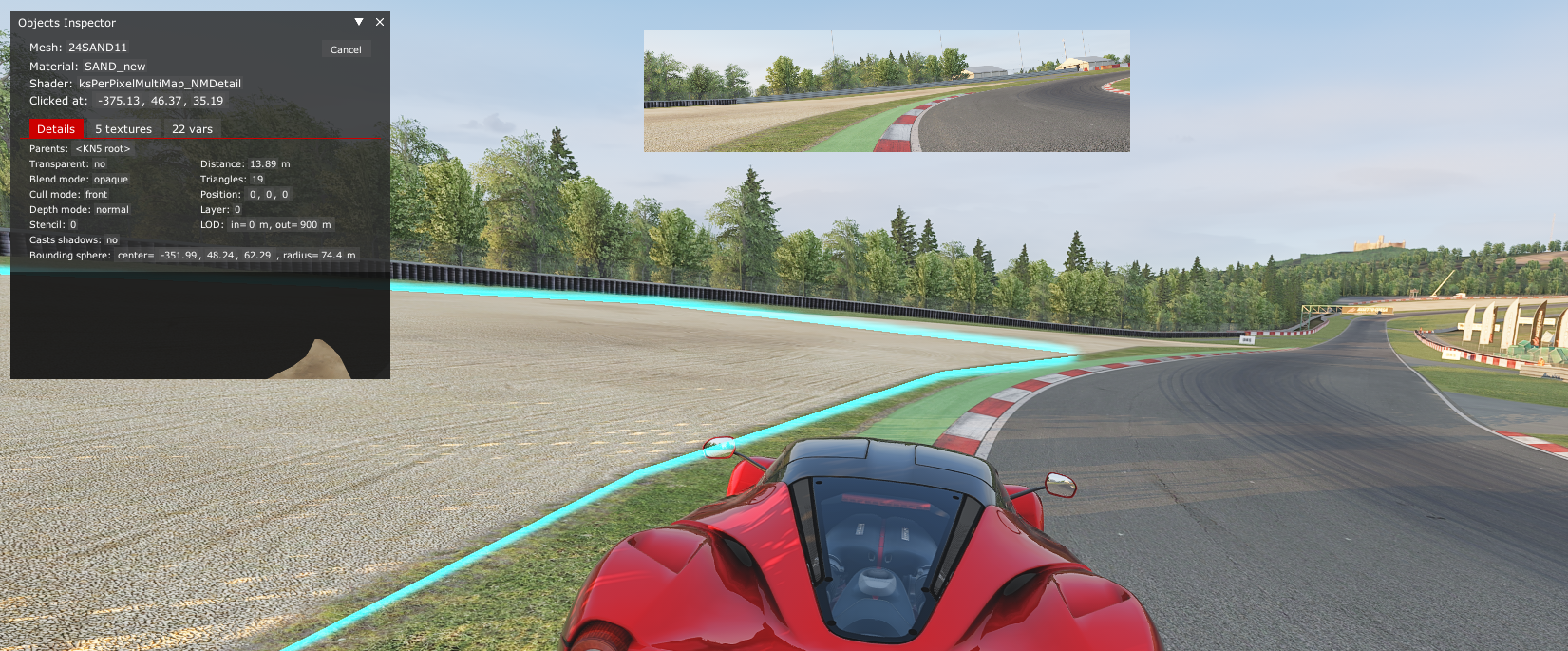Some tracks in AC don't report cuts to the server properly in race sessions. This won't matter if you're not doing anything with cuts or if you're running an external application (such as Pit Lane Penalty) to track them. But if you want to keep track of race cuts using the server only you need to make the following modifications to the offending track's `surfaces.ini` file!

1. If `BLACK_FLAG_TIME` is set to 0 then invalid track surfaces won't register cuts in race sessions, but will in practice/qualifying

2. Some tracks are missing certain surfaces from `surfaces.ini` that should be there. I have no idea why these surfaces register cuts in practice/qualifying by default, but for races they need adding! Have a look at the example below for more details on this and how to find these surfaces.

I'm going to go through the process of fixing a track for cuts in a race session (on a server), lets have a look at Nurburgring Sprint B. First I'm going to have a look at the `surfaces.ini` file and replace any instances of `BLACK_FLAG_TIME=0` with `BLACK_FLAG_TIME=5`. There were a few that had this issue! Here is an example:

``````[SURFACE_5]
KEY=OUT
FRICTION=0.95
DAMPING=0.01
WAV=kerb.wav
WAV_PITCH=1.2
FF_EFFECT=1
BLACK_FLAG_TIME=5 // this OUT surface needed this value modifying from 0 to 5
IS_VALID_TRACK=0
SIN_HEIGHT=0
SIN_LENGTH=0
IS_PITLANE=0
VIBRATION_GAIN=1.0
VIBRATION_LENGTH=1.0
``````

Now I'm going to boot the game and look around for any surfaces that it looks like you could cut across that either aren't in `surfaces.ini` at all or are set as valid track surfaces. In order to do this I'm using the "Objects Inspector" app included with Shaders Patch/Content Manager.And at the first corner we find our first offender! This bit of grass has the mesh `GRASS` set to it (you can ignore the numbers here), and is an obvious place to try and cut the track. `GRASS` is missing completely from the default `surfaces.ini` file (and most corners have this mesh on the inside!), so we need to add it.

Please note that using the "mesh" name here is an assumption, the mesh name may not always match the surface name but it usually does! If the mesh name doesn't work try the material name, and if that doesn't work unfortunately I think your best guess is the only option (for example Mugello has all sorts of odd names for the grass mesh/material, but the surface is called `GRASS` as it usually is).

``````[SURFACE_10]
KEY=GRASS
FRICTION=0.6
DAMPING=0
WAV=grass.wav
WAV_PITCH=0
FF_EFFECT=NULL
IS_VALID_TRACK=0
BLACK_FLAG_TIME=5
SIN_HEIGHT=0.03
SIN_LENGTH=0.5
IS_PITLANE=0
VIBRATION_GAIN=0.2
VIBRATION_LENGTH=0.6
``````

I copied the values for `FRICTION` etc. from the `GRS-CUT-B` surface included in this `surfaces.ini`. Coincidentally I couldn't find any `GRS-CUT-B` meshes on the track anywhere! Perhaps the intention was to change some of the meshes to this at a later date, but it was never done, who knows! If I couldn't find a set of values for grass in this file I'd have just had a look through my other track's `surfaces.ini` files until I found one.At turn three I found a mesh on the inside called `CUTKRB`, this mesh is already included as a surface in the `surfaces.ini`, it is `SURFACE_3`. Nice to see, we can move on!This one is a bit questionable, and probably up to your ideas of what a cut should be, remember just because it's off the track doesn't mean it has to be a cut! This `SAND` mesh is on the outside of turn 7 (I think) and if somebody hits it they're definitely having a bad time, and have probably crossed a `GRASS` mesh to get there anyway. But for the sake of completeness I'll add a `SAND` surface to `surfaces.ini`

``````[SURFACE_11]
KEY=SAND
FRICTION=0.8
DAMPING=0.1
WAV=sand.wav
WAV_PITCH=0
FF_EFFECT=0
IS_VALID_TRACK=0
BLACK_FLAG_TIME=5
SIN_HEIGHT=0.04
SIN_LENGTH=0.5
IS_PITLANE=0
VIBRATION_GAIN=0.2
VIBRATION_LENGTH=0.3
``````

It's important to note that when I add a new surface I'm incrementing the surface number from the current last surface in the file, for example `SURFACE_11` here, don't overlook that, it's important!

And that's it! All cuts around the Nurburgring Sprint B track are now properly reported in race sessions! I'll pop the full final `surfaces.ini` file below for this track.

If you want to modify a track like this you need to then share the updated `surfaces.ini` file with your drivers! I'd actually suggest making a copy of the track, renaming it (rename the main folder, the main kn5 file and any models.ini files to point at the new kn5 name) and then sharing that! That way your drivers don't need to worry about making backups of their `surface.ini` files in order to play on other servers.

Full fixed file:

``````[SURFACE_0]
KEY=CONCRETE
FRICTION=0.85
DAMPING=0
WAV=
WAV_PITCH=0
FF_EFFECT=NULL
BLACK_FLAG_TIME=0
IS_VALID_TRACK=1
SIN_HEIGHT=0
SIN_LENGTH=0
IS_PITLANE=0
VIBRATION_GAIN=0
VIBRATION_LENGTH=0

[SURFACE_1]
KEY=CARPET
FRICTION=0.75
DAMPING=0
WAV=extraturf.wav
WAV_PITCH=0
FF_EFFECT=NULL
BLACK_FLAG_TIME=0
IS_VALID_TRACK=1
SIN_HEIGHT=0
SIN_LENGTH=0
IS_PITLANE=0
VIBRATION_GAIN=0
VIBRATION_LENGTH=0

[SURFACE_2]
KEY=PITS
FRICTION=0.95
DAMPING=0
WAV=
WAV_PITCH=0
FF_EFFECT=NULL
BLACK_FLAG_TIME=0
IS_VALID_TRACK=1
SIN_HEIGHT=0
SIN_LENGTH=0
IS_PITLANE=1
VIBRATION_GAIN=0
VIBRATION_LENGTH=0

[SURFACE_3]
KEY=CUTKRB
FRICTION=0.95
DAMPING=0.01
WAV=kerb.wav
WAV_PITCH=1.2
FF_EFFECT=1
BLACK_FLAG_TIME=5
IS_VALID_TRACK=0
SIN_HEIGHT=0
SIN_LENGTH=0
IS_PITLANE=0
VIBRATION_GAIN=1.0
VIBRATION_LENGTH=1.0

[SURFACE_4]
KEY=PAINT
FRICTION=0.92
DAMPING=0
WAV=
WAV_PITCH=0
FF_EFFECT=NULL
BLACK_FLAG_TIME=0
IS_VALID_TRACK=1
SIN_HEIGHT=0
SIN_LENGTH=0
IS_PITLANE=0
VIBRATION_GAIN=0
VIBRATION_LENGTH=0

[SURFACE_5]
KEY=OUT
FRICTION=0.95
DAMPING=0.012
WAV=
WAV_PITCH=0
FF_EFFECT=NULL
BLACK_FLAG_TIME=5
IS_VALID_TRACK=0
SIN_HEIGHT=0
SIN_LENGTH=0
IS_PITLANE=0
VIBRATION_GAIN=0
VIBRATION_LENGTH=0

[SURFACE_6]
KEY=ASPH-NURB
FRICTION=0.99
DAMPING=0
WAV=
WAV_PITCH=0
FF_EFFECT=NULL
BLACK_FLAG_TIME=0
IS_VALID_TRACK=1
SIN_HEIGHT=0
SIN_LENGTH=0
IS_PITLANE=0
VIBRATION_GAIN=0
VIBRATION_LENGTH=0

[SURFACE_7]
KEY=CURB
FRICTION=0.85
DAMPING=0
WAV=kerb.wav
WAV_PITCH=1.3
FF_EFFECT=1
BLACK_FLAG_TIME=0
IS_VALID_TRACK=1
SIN_HEIGHT=0
SIN_LENGTH=0
IS_PITLANE=0
VIBRATION_GAIN=1.0
VIBRATION_LENGTH=1.0

[SURFACE_8]
KEY=GRS-CUT-B
FRICTION=0.6
DAMPING=0
WAV=grass.wav
WAV_PITCH=0
FF_EFFECT=NULL
IS_VALID_TRACK=0
BLACK_FLAG_TIME=5
SIN_HEIGHT=0.03
SIN_LENGTH=0.5
IS_PITLANE=0
VIBRATION_GAIN=0.2
VIBRATION_LENGTH=0.6

[SURFACE_9]
KEY=TRM-CUT-A
FRICTION=0.99
DAMPING=0.012
WAV=
WAV_PITCH=0
FF_EFFECT=NULL
BLACK_FLAG_TIME=5
IS_VALID_TRACK=0
SIN_HEIGHT=0
SIN_LENGTH=0
IS_PITLANE=0
VIBRATION_GAIN=0
VIBRATION_LENGTH=0

[SURFACE_10]
KEY=GRASS
FRICTION=0.6
DAMPING=0
WAV=grass.wav
WAV_PITCH=0
FF_EFFECT=NULL
IS_VALID_TRACK=0
BLACK_FLAG_TIME=5
SIN_HEIGHT=0.03
SIN_LENGTH=0.5
IS_PITLANE=0
VIBRATION_GAIN=0.2
VIBRATION_LENGTH=0.6

[SURFACE_11]
KEY=SAND
FRICTION=0.8
DAMPING=0.1
WAV=sand.wav
WAV_PITCH=0
FF_EFFECT=0